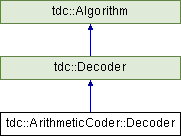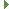tudocomp – The TU Dortmund Compression Framework
tdc::ArithmeticCoder::Decoder Class Reference

Decodes data from an Arithmetic character stream. More...

`#include <ArithmeticCoder.hpp>`

Inheritance diagram for tdc::ArithmeticCoder::Decoder:## Public Member Functions

DECODER_CTOR (env, in)

template<typename value_t >
value_t decode (const LiteralRange &)Public Member Functions inherited from tdc::Decoder
Decoder (Env &&env, std::shared_ptr< BitIStream > in)
Constructor. More...

Decoder (Env &&env, Input &in)
Convenience constructor. More...

bool eof () const
Tests whether the end of the bit input stream has been reached. More...

template<typename value_t >
value_t decode (const Range &r)
Decodes an arbitrary-range integer value. More...

template<typename value_t >
value_t decode (const BitRange &r)
Decodes a bit. More...

const std::shared_ptr< BitIStream > & stream ()Public Member Functions inherited from tdc::Algorithm
virtual ~Algorithm ()=default

Algorithm (Algorithm const &)=default

Algorithm (Algorithm &&)=default

Algorithmoperator= (Algorithm const &)=default

Algorithmoperator= (Algorithm &&)=default

Algorithm (Env &&env)
Instantiates an algorithm in the specified environment. More...

Envenv ()

const Envenv () constProtected Attributes inherited from tdc::Decoder
std::shared_ptr< BitIStreamm_in
The underlying bit input stream. More...

## Detailed Description

Decodes data from an Arithmetic character stream.

Definition at line 180 of file coders/ArithmeticCoder.hpp.

## ◆ decode()

template<typename value_t >
 value_t tdc::ArithmeticCoder::Decoder::decode ( const LiteralRange & )
inline

Definition at line 243 of file coders/ArithmeticCoder.hpp.

## ◆ DECODER_CTOR()

 tdc::ArithmeticCoder::Decoder::DECODER_CTOR ( env , in )
inline

Definition at line 224 of file coders/ArithmeticCoder.hpp.

The documentation for this class was generated from the following file: